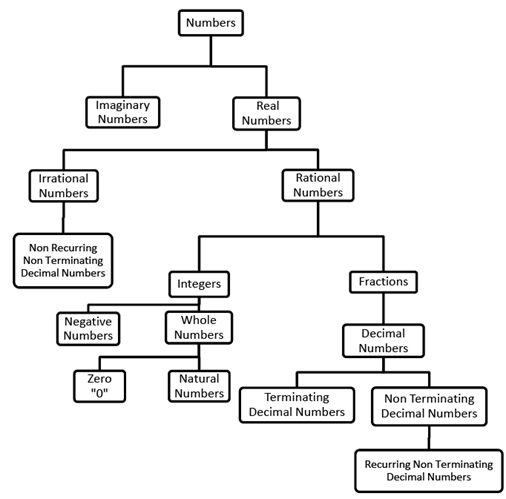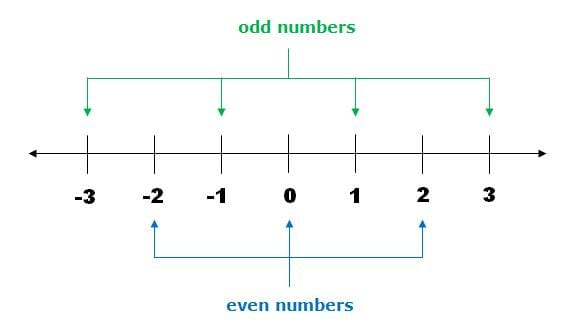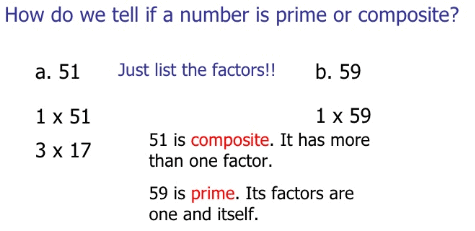UPSC  >  Important Concepts: Number System - 2

# Important Concepts: Number System - 2 Notes | Study CSAT Preparation for UPSC CSE - UPSC

## Document Description: Important Concepts: Number System - 2 for UPSC 2022 is part of CSAT Preparation for UPSC CSE preparation. The notes and questions for Important Concepts: Number System - 2 have been prepared according to the UPSC exam syllabus. Information about Important Concepts: Number System - 2 covers topics like The Number Tree and Important Concepts: Number System - 2 Example, for UPSC 2022 Exam. Find important definitions, questions, notes, meanings, examples, exercises and tests below for Important Concepts: Number System - 2.

Introduction of Important Concepts: Number System - 2 in English is available as part of our CSAT Preparation for UPSC CSE for UPSC & Important Concepts: Number System - 2 in Hindi for CSAT Preparation for UPSC CSE course. Download more important topics related with notes, lectures and mock test series for UPSC Exam by signing up for free. UPSC: Important Concepts: Number System - 2 Notes | Study CSAT Preparation for UPSC CSE - UPSC
 1 Crore+ students have signed up on EduRev. Have you?

The Number Tree10. Odd and Even Numbers• This is a property of Integers. But since our questions are limited to the Whole numbers, we will keep our studies limited to them.
• By Definition, Even Numbers are those which are divisible by 2. They are the numbers with their unit digit as either 2, 4, 6, 8 or 0. The general expression of Even numbers is 2n where n is a whole number.
• By Definition, Odd Numbers are those which are not divisible by 2. They are the numbers with their unit digit as either 1, 3, 5, 7 or 9. The general expression of Odd numbers is 2n+1 where n is a whole number.

Certain Operations on Whole Numbers:
(i) Odd ± Odd = Even
(ii) Even ± Even = Even
(iii) Odd ± Even = Odd
(iv) Even ± Even ± Even ........... n terms = Even
(v) Odd ± Odd ± Odd .............. n terms = Even if n is Even, Odd if n is Odd
(vi) Odd × Odd = Odd
(vii) Even × Odd = Even
(viii) Even × Even = Even
(ix) Even × Even × Even ....... n terms = Even
(x) Odd × Odd × Odd ....... n terms = Odd
(xi) (Even)n = Even
(xii) (odd)n = Odd

Question for Important Concepts: Number System - 2
Try yourself:If a is even what would 5a + 1 be

Question for Important Concepts: Number System - 2
Try yourself:If a is even and b is odd what would a3 + 3b + 6 be

Question for Important Concepts: Number System - 2
Try yourself:If 5a - 2 is even what would a  be

11. Prime and Composite Numbers

(a) Prime Numbers: Being Prime is a property of Natural Numbers. All those numbers which are divisible by exactly 2 numbers, i.e. itself and unity "1".
Example: 2, 3, 5, 7, 11 etc.

(b) Composite are the numbers which are divisible by more than 2 numbers, i.e. itself, unity "1" and at least one other.
Example: 4, 6, 8, 9, 10 etc.

1 is neither Prime nor Composite.Question for Important Concepts: Number System - 2
Try yourself:How many Prime numbers are there less than 100

Question for Important Concepts: Number System - 2
Try yourself:Which of the following cannot be the sum of two prime numbers.

Question for Important Concepts: Number System - 2
Try yourself:State Whether True or False

All Prime numbers are Odd

Question for Important Concepts: Number System - 2
Try yourself:State Whether True or False

Sum of Any 2 prime numbers is always Even

Question for Important Concepts: Number System - 2
Try yourself:State Whether True or False

Product of Any two prime numbers is always Odd

Question for Important Concepts: Number System - 2
Try yourself:State Whether True or False

There are no 4 digit Prime numbers.

Property of Prime Numbers

• All Prime numbers greater than 3 are of the form 6n ± 1 but not all numbers of form 6n ± 1 are Prime. This property means when prime numbers greater than 3 are divided by 6 they leave a remainder of 1 or 5.

Question for Important Concepts: Number System - 2
Try yourself:Which of the following is a prime numbers.

12. Co - Prime Numbers

• This is a property of a pair or more than two natural numbers.
• Two numbers are said to be co-prime if the only number which can completely divide both the numbers is 1. No number other than 1 can divide both the given numbers of the pair.
• These numbers in themselves can be prime or composite. 1 is co-prime with all the natural numbers other than 1.
Example: (3, 7); (4, 15); (3, 10) etc.
• A set of numbers is said to be co-prime if their HCF is 1. We will be learning about HCF in the following chapters.
Example: (3, 7, 11); (4, 15, 10); (3, 10, 8) etc.
The document Important Concepts: Number System - 2 Notes | Study CSAT Preparation for UPSC CSE - UPSC is a part of the UPSC Course CSAT Preparation for UPSC CSE.
All you need of UPSC at this link: UPSC

## CSAT Preparation for UPSC CSE

72 videos|64 docs|92 tests
 Use Code STAYHOME200 and get INR 200 additional OFF

## CSAT Preparation for UPSC CSE

72 videos|64 docs|92 tests

### How to Prepare for UPSC

Read our guide to prepare for UPSC which is created by Toppers & the best Teachers

Track your progress, build streaks, highlight & save important lessons and more!

,

,

,

,

,

,

,

,

,

,

,

,

,

,

,

,

,

,

,

,

,

;